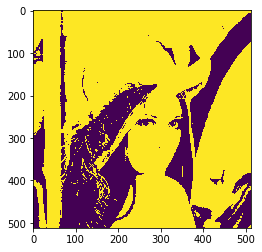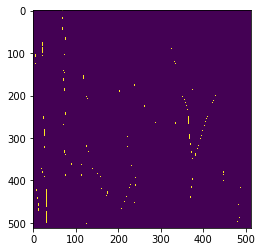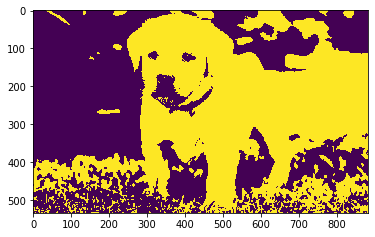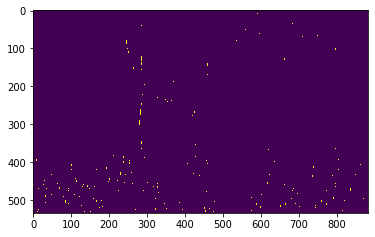Related Articles
Mahotas – Match template to image exactly
• Last Updated : 10 Jul, 2020

In this article we will see how we can match a template with the image in mahotas. By matching templates we can find the specific location or things in a image with the help of structure we want to find.

In this tutorial we will use “lena” image, below is the command to load it.

```mahotas.demos.load('lena')
```

Below is the lena imageIn order to do this we will use `mahotas.find` method

Syntax : mahotas.find(img, template)

Argument : It takes image object and template as argument

Return : It returns boolean ndarray

Note : Input image should be filtered or should be loaded as grey

In order to filter the image we will take the image object which is numpy.ndarray and filter it with the help of indexing, below is the command to do this

`image = image[:, :, 0]`

Below is the implementation

 `# importing required libraries ` `import` `mahotas ` `import` `mahotas.demos ` `from` `pylab ``import` `gray, imshow, show ` `import` `numpy as np ` `   `  `# loading iamge ` `img ``=` `mahotas.demos.load(``'lena'``) ` ` `  ` `  `   `  `# filtering image ` `img ``=` `img.``max``(``2``) ` ` `  `# otsu method ` `T_otsu ``=` `mahotas.otsu(img)    ` `   `  `# image values should be greater than otsu value ` `img ``=` `img > T_otsu ` `   `  `print``(``"Image threshold using Otsu Mehtod"``) ` ` `  `# showing image ` `imshow(img) ` `show() ` ` `  `# template ` `template ``=` `np.array([ ` `        ``[``0``, ``1``, ``1``], ` `        ``[``0``, ``1``, ``1``], ` `        ``[``0``, ``1``, ``1``]], ``bool``) ` ` `  ` `  `# finding template in the image ` `new_img ``=` `mahotas.find(img, template) ` ` `  `print``(``"Image with only templates"``) ` `# showing new image ` `imshow(new_img) ` `show() `

Output :

`Image threshold using Otsu Mehtod``Image with only templates`Another example

 `# importing required libraries ` `import` `mahotas ` `import` `numpy as np ` `from` `pylab ``import` `gray, imshow, show ` `import` `os ` `  `  `# loading iamge ` `img ``=` `mahotas.imread(``'dog_image.png'``) ` ` `  ` `  `# fltering image ` `img ``=` `img[:, :, ``0``] ` `   `  `# otsu method ` `T_otsu ``=` `mahotas.otsu(img)    ` `   `  `# image values should be greater than otsu value ` `img ``=` `img > T_otsu ` `   `  `print``(``"Image threshold using Otsu Mehtod"``) ` `   `  `# showing image ` `imshow(img) ` `show() ` ` `  `# template ` `template ``=` `np.array([ ` `        ``[``0``, ``1``, ``1``], ` `        ``[``0``, ``1``, ``1``], ` `        ``[``0``, ``1``, ``1``]], ``bool``) ` ` `  ` `  `# finding template in the image ` `new_img ``=` `mahotas.find(img, template) ` ` `  `print``(``"Image with only templates"``) ` `# showing new image ` `imshow(new_img) ` `show() `

Output :

`Image threshold using Otsu Mehtod``Image with only templates`Attention geek! Strengthen your foundations with the Python Programming Foundation Course and learn the basics.

To begin with, your interview preparations Enhance your Data Structures concepts with the Python DS Course.

My Personal Notes arrow_drop_up
Recommended Articles
Page :